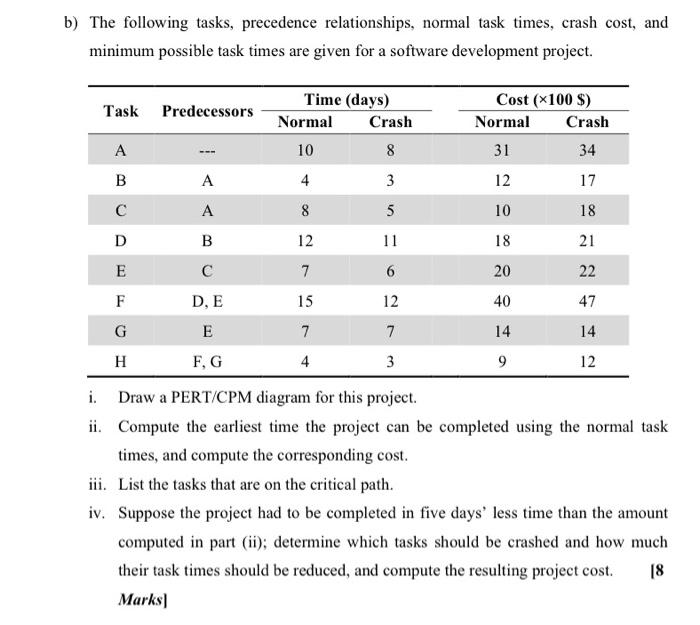# (Solved): b) The following tasks, precedence relationships, normal task times, crash cost, and minimum possib ...b) The following tasks, precedence relationships, normal task times, crash cost, and minimum possible task times are given for a software development project. i. Draw a PERT/CPM diagram for this project. ii. Compute the earliest time the project can be completed using the normal task times, and compute the corresponding cost. iii. List the tasks that are on the critical path. iv. Suppose the project had to be completed in five days' less time than the amount computed in part (ii); determine which tasks should be crashed and how much their task times should be reduced, and compute the resulting project cost. \( [8 \) Marks]

We have an Answer from Expert